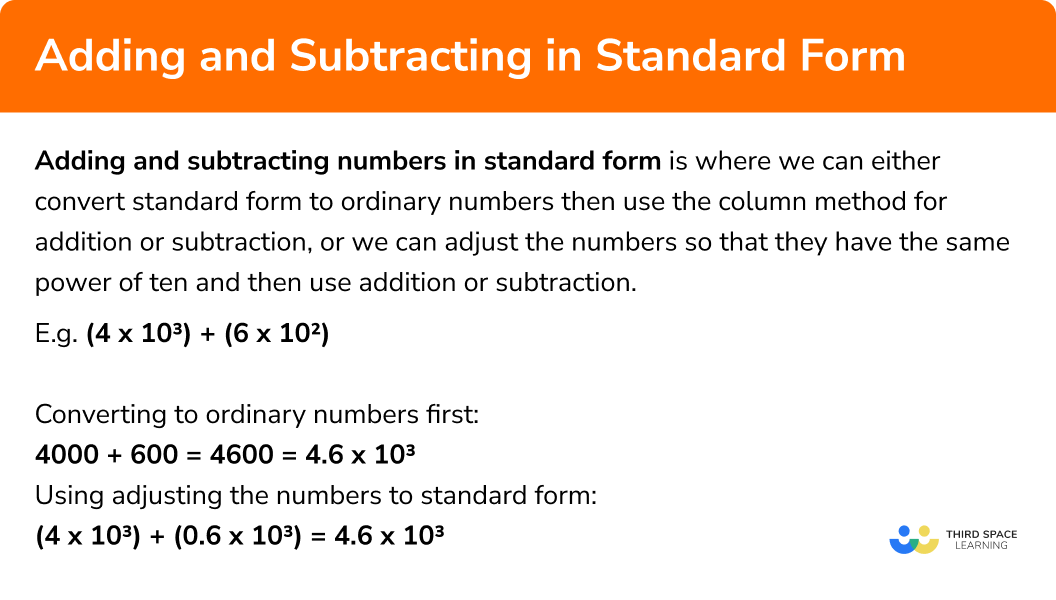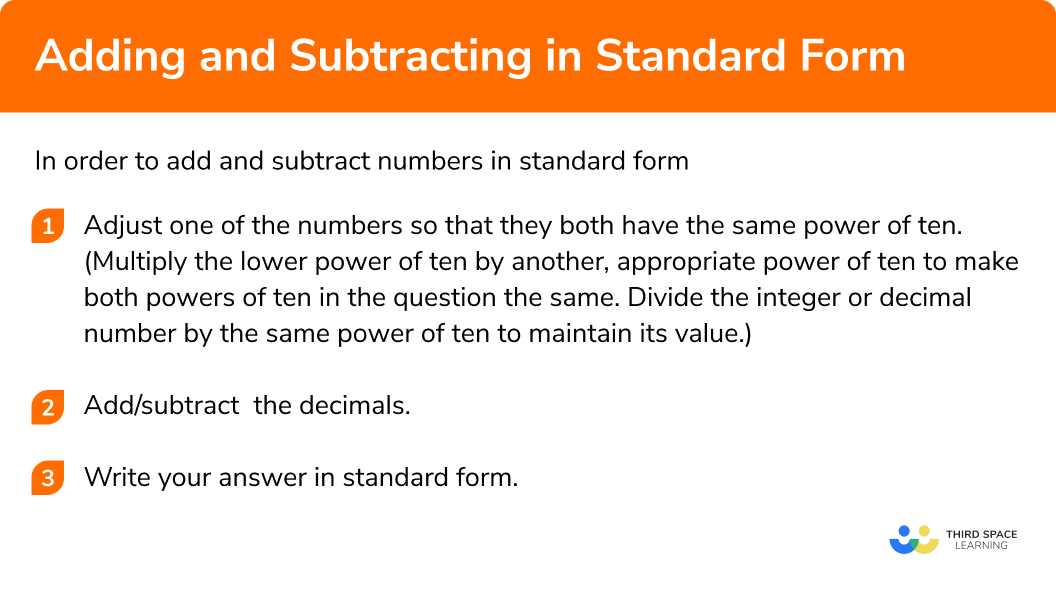GCSE Maths Number Standard Form

Adding and Subtracting in Standard Form

# Adding and Subtracting in Standard Form

Here we will learn about adding and subtracting numbers in standard form.

There are also adding and subtracting numbers in standard form worksheets based on Edexcel, AQA and OCR exam questions, along with further guidance on where to go next if you’re still stuck.

## What is adding and subtracting in standard form?

Adding and subtracting in standard form works in a similar way to adding and subtracting ordinary numbers. There are two methods we can use.

We can either convert standard form to ordinary numbers then use the column method for addition or subtraction, or we can adjust the numbers so that they have the same power of ten and then use addition or subtraction.

E.g.
(4\times10^{3})+(6\times10^{2})

Converting to ordinary numbers first:  4000 + 600 = 4600
However, this method is not very efficient especially for very large and very small numbers.

To add and subtract numbers in standard we can first convert the numbers so that they have the same power of ten.

E.g.
Using standard form: (4\times10^{3})+(0.6\times10^{3})=(4.6\times10^{3})

### What is adding and subtracting in standard form?## How to add and subtract with standard form

In order to add and subtract numbers in standard form:

1. Convert one of the numbers so that both numbers have the same power of ten. Select the number with the lower power of 10.

### How to add and subtract with standard form## Adding and subtracting standard form examples

### Example 1: adding numbers in standard form

Work out:

$(5\times10^{5})\quad+\quad(2\times10^{4})$

1. Convert one of the numbers so that both numbers have the same power of ten. Select the number with the lower power of 10.

10^{4} is the lowest power of ten.  Multiply it by 10 so it also becomes 10^{5}

10^{4} x 1= 10^{5}

Divide 2 by 10 to maintain the value:

2 ÷ 10 = 0.2

2 \times 10^{4} = 0.2 \times 10^{5}

2 Add & subtract the decimals.

5 + 0.2 = 5.2

( 5 x 10^{5}) + ( 0.2 x 10^{5}) = 5.2 x 10^{5}

### Example 2: adding numbers in standard form

Work out:

$(7\times10^{-3})\quad+\quad(6\times10^{-4})$

Convert one of the numbers so that both numbers have the same power of ten. Select the number with the lower power of 10.

### Example 3: adding numbers in standard form

Work out

$(8.1\times10^{7})\quad+\quad(2.5\times10^{5})$

Convert one of the numbers so that both numbers have the same power of ten. Select the number with the lower power of 10.

### Example 4: subtracting numbers in standard form

Calculate

$(8\times10^{4})\quad-\quad(6\times10^{3})$

Convert one of the numbers so that both numbers have the same power of ten. Select the number with the lower power of 10.

### Example 5: subtracting numbers in standard form

Calculate

$(8\times10^{-2})\quad-\quad(3\times10^{-3})$

Convert one of the numbers so that both numbers have the same power of ten. Select the number with the lower power of 10.

### Example 6: subtracting numbers in standard form

Calculate

$(6.2\times10^{4})\quad-\quad(1.8\times10^{2})$

Convert one of the numbers so that both numbers have the same power of ten. Select the number with the lower power of 10.

### Common misconceptions

• Using the column method

While this is not wrong, it is an inefficient method and can lead to errors when converting the numbers, especially with very large and very small numbers with many place holders.

E.g.

$8000 + 600 = 8600 = 8.6\times10^{4}$

Using standard form:

$(8\times10^{4})\quad+\quad(0.6\times10^{4})\quad=\quad8.6\times10^{4}$

• Not converting solutions to standard form

After calculating with standard form, a common mistake is not ensuring the number is in standard form.
Remember to be in standard form the number needs to have two parts, the first part should between 1 and 10 (1n < 10) and the second part should be a power of 10.

E.g.
62\times10^{7} is not in standard form as 62 is greater than 10.
In standard for this should be written as 6.2\times10^{8}

• Negative powers

A common mistake is to become mixed up when using negative powers.
E.g.
10^{-3}  is smaller than 10^{-2} because -3 is less than -2 .
10^{-3}=0.001 and 10^{-2}=0.01 so 10^{-2} is greater than 10^{-3}

Adding and subtracting standard form is part of our series of lessons to support revision on standard form. You may find it helpful to start with the main standard form lesson for a summary of what to expect, or use the step by step guides below for further detail on individual topics. Other lessons in this series include:

### Practice adding standard form and subtracting standard form questions

23 \times 10^{5}5\times 10^{11}3.2 \times 10^{5}2.3 \times 10^{6}3 \times 10^{5}=0.3 \times 10^{6}\\ (2 \times 10^{6})+(0.3 \times 10^{6}=2.3 \times 10^{6}

4.7 \times 10^{-5}4.7 \times 10^{-4}7.4 \times 10^{-4}47 \times 10^{-6}7 \times 10^{-6}=0.7 \times 10^{-5}\\ (4 \times 10^{-5}) + (0.7 \times 10^{-5})=4.7 \times 10^{-5}

6.75 \times 10^{8}643.5 \times 10^{6}6.435 \times 10^{8}9.9 \times 10^{14}3.5 \times 10^{6} = 0.035 \times 10^{8}\\ (6.4 \times 10^{8}) + (0.035 \times 10^{8}) = 6.435 \times 10^{8}

7.4 \times 10^{4}66 \times 10^{3}6.6 \times 10^{4}3 \times 10^{4}4 \times 10^{3} = 0.4 \times 10^{4}\\ (7 \times 10^{4})-(0.4 \times 10^{4})=6.6 \times 10^{4}

4.8 \times 10^{-3}1.5 \times 10^{-3}4.8 \times 10^{-4}3 \times 10^{1}2 \times 10^{-4} = 0.2 \times 10^{-3}\\ (5 \times 10^{-3})-(0.2 \times 10^{-3}) = 4.8 \times 10^{-3}

7.57 \times 10^{5}7.823 \times 10^{5}777.7 \times 10^{3}7.777 \times 10^{5}2.3 \times 10^{3} = 0.023 \times 10^{5}\\ (7.8 \times 10^{5})-(0.023 \times 10^{5}) = 7.777 \times 10^{5}

### Adding and subtracting standard form GCSE questions

1. The table below shows the population of several cities

 City Population London 8.9 x 10^6 Manchester 5.5 x 10^5 Birmingham 1.1 x 10^6 Oxford 1.1 x 10^5

Work out the total population of London and Manchester. Give your answer in standard form.

(3 marks)

Simplifying by writing the two populations as an ordinary number:
London: 8900000
Manchester: 550000

OR

converting 5.5\times10^{5} to 0.55\times10^{6}

(1)

8900000 + 550000 = 9450000

OR

8.9\times10^{6} +0.55\times10^{6}  = 9.45 \times 10^{6}

(1)

9.45 \times 10^{6}

(1)

(3 marks)

Simplifying by writing the two numbers as an ordinary numbers:

7000000 – 400000

OR

converting 4\times10^{5} to 0.4\times10^{6}

(1)

Subtracting the numbers:
7000000 – 400000 = 6600000

OR

7\times10^{6} – 0.4\times10^{6}  = 6.6 \times 10^{6}

(1)

6.6\times10^{6}

(1)

3. Show that

(2 marks)

240 + 3700 or 0.24\times10^{3} or 39.4\times10^{2}

(1)

Correct working shown

(1)

## Learning checklist

You have now learned how to:

• Add numbers in standard form
• Subtract numbers in standard form

## Still stuck?

Prepare your KS4 students for maths GCSEs success with Third Space Learning. Weekly online one to one GCSE maths revision lessons delivered by expert maths tutors.

Find out more about our GCSE maths tuition programme.